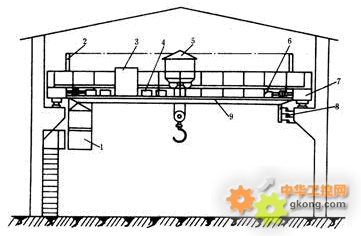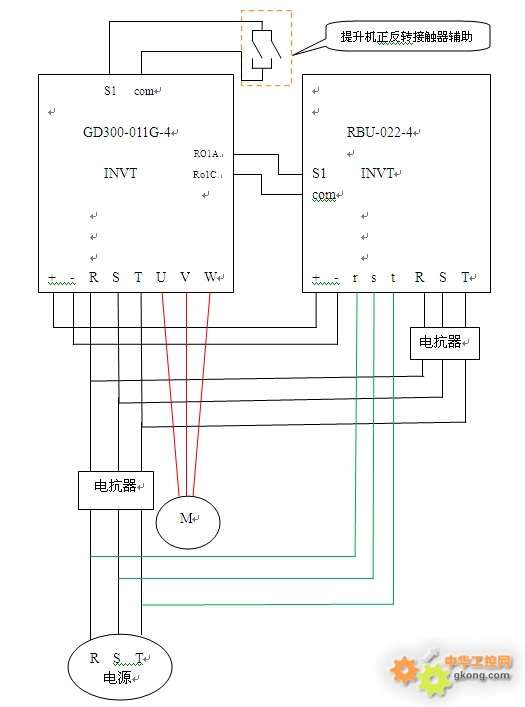# 英威腾GD300变频器加能量回馈单元在行车上的应用

2016/8/26 9:29:241．桥架：由主梁。端梁。走台等几部分组成。

2．大车移行机构。

3．小车。

4．提升机构。

5．操纵室。P00.01=1（端子运行指令）

P00.11=1（加速时间）

P00.12=1（减速时间）

P00.04=45（运行频上限）

P00.06=0（频率由键盘数字设定）

P00.10=45 (键盘设定频率)

P01.01=4（直接起动开始频率）

P01.08=1（自由停车）

P08.28=4  (自动复位次数)

RBU参数设定：

P0.00=1（端子控制）

P0.02=4 （开关量虑波次数）

P0.20=3 （自动复位次数）

CHE100在空压机上的应用

CHV100变频器在卷染机上的应用

CHF100变频器用于中央空调节能改造

DA200伺服在飞剪的应用